# module mlmodel.quantile_regression#

## Short summary#

module mlinsights.mlmodel.quantile_regression

Implements a quantile linear regression.

source on GitHub

## Classes#

class

truncated documentation

QuantileLinearRegression

Quantile Linear Regression or linear regression trained with norm L1. This class inherits from sklearn.linear_models.LinearRegression. …

## Properties#

property

truncated documentation

_repr_html_

HTML representation of estimator. This is redundant with the logic of _repr_mimebundle_. The latter should …

## Static Methods#

staticmethod

truncated documentation

_epsilon

## Methods#

method

truncated documentation

__init__

fit

Fits a linear model with L1 norm which is equivalent to a quantile regression. The implementation …

score

Returns Mean absolute error regression loss.

## Documentation#

Implements a quantile linear regression.

source on GitHub

class mlinsights.mlmodel.quantile_regression.QuantileLinearRegression(fit_intercept=True, copy_X=True, n_jobs=1, delta=0.0001, max_iter=10, quantile=0.5, positive=False, verbose=False)#

Quantile Linear Regression or linear regression trained with norm L1. This class inherits from sklearn.linear_models.LinearRegression. See notebook Quantile Regression.

Norm L1 is chosen if quantile=0.5, otherwise, for quantile=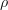, the following error is optimized: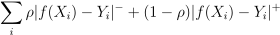where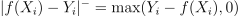and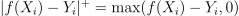.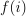is the prediction,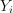the expected value.

source on GitHub

Parameters:
• fit_intercept – boolean, optional, default True whether to calculate the intercept for this model. If set to False, no intercept will be used in calculations (e.g. data is expected to be already centered).

• copy_X – boolean, optional, default True If True, X will be copied; else, it may be overwritten.

• n_jobs – int, optional, default 1 The number of jobs to use for the computation. If -1 all CPUs are used. This will only provide speedup for n_targets > 1 and sufficient large problems.

• max_iter – int, optional, default 1 The number of iteration to do at training time. This parameter is specific to the quantile regression.

• delta – float, optional, default 0.0001 Used to ensure matrices has an inverse (M + delta*I).

• quantile – float, by default 0.5, determines which quantile to use to estimate the regression.

• positive – when set to True, forces the coefficients to be positive.

• verbose – bool, optional, default False Prints error at each iteration of the optimisation.

source on GitHub

__abstractmethods__ = frozenset({})#
__init__(fit_intercept=True, copy_X=True, n_jobs=1, delta=0.0001, max_iter=10, quantile=0.5, positive=False, verbose=False)#
Parameters:
• fit_intercept – boolean, optional, default True whether to calculate the intercept for this model. If set to False, no intercept will be used in calculations (e.g. data is expected to be already centered).

• copy_X – boolean, optional, default True If True, X will be copied; else, it may be overwritten.

• n_jobs – int, optional, default 1 The number of jobs to use for the computation. If -1 all CPUs are used. This will only provide speedup for n_targets > 1 and sufficient large problems.

• max_iter – int, optional, default 1 The number of iteration to do at training time. This parameter is specific to the quantile regression.

• delta – float, optional, default 0.0001 Used to ensure matrices has an inverse (M + delta*I).

• quantile – float, by default 0.5, determines which quantile to use to estimate the regression.

• positive – when set to True, forces the coefficients to be positive.

• verbose – bool, optional, default False Prints error at each iteration of the optimisation.

source on GitHub

_abc_impl = <_abc._abc_data object>#
static _epsilon(y_true, y_pred, quantile, sample_weight=None)#
fit(X, y, sample_weight=None)#

Fits a linear model with L1 norm which is equivalent to a quantile regression. The implementation is not the most efficient as it calls multiple times method fit from sklearn.linear_models.LinearRegression. Data gets checked and rescaled each time. The optimization follows the algorithm Iteratively reweighted least squares. It is described in French at Régression quantile.

Parameters:
• X – numpy array or sparse matrix of shape [n_samples,n_features] Training data

• y – numpy array of shape [n_samples, n_targets] Target values. Will be cast to X’s dtype if necessary

• sample_weight – numpy array of shape [n_samples] Individual weights for each sample

Returns:

self, returns an instance of self.

Fitted attributes:

• coef_: array, shape (n_features, ) or (n_targets, n_features)

Estimated coefficients for the linear regression problem. If multiple targets are passed during the fit (y 2D), this is a 2D array of shape (n_targets, n_features), while if only one target is passed, this is a 1D array of length n_features.

• intercept_: array

Independent term in the linear model.

• n_iter_: int

Number of iterations at training time.

source on GitHub

score(X, y, sample_weight=None)#

Returns Mean absolute error regression loss.

Parameters:
• X – array-like, shape = (n_samples, n_features) Test samples.

• y – array-like, shape = (n_samples) or (n_samples, n_outputs) True values for X.

• sample_weight – array-like, shape = [n_samples], optional Sample weights.

Returns:

score : float mean absolute error regression loss

source on GitHub